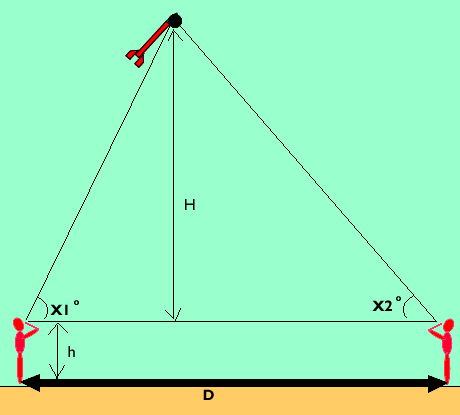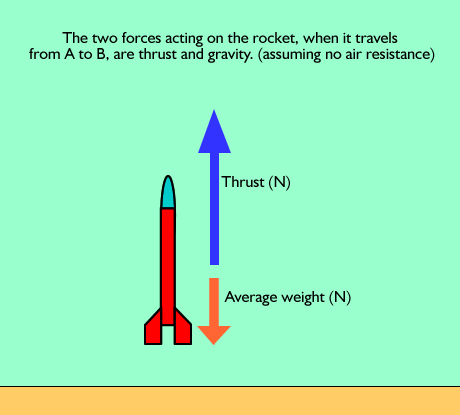Rocket activity Original worksheet was produced by Philip AnthonyDEFINITION OF TERMS Maximum height of rocket = H + h Mass of rocket before firing = mi Mass of rocket after fuel is exhausted = mf Time from A to C (stopwatch) = tAC Time from A to B = tAB Time from B to C = tBC Average drag from B to C = FBC Vertical component of AB = xAB Vertical component of BC = xBC Distance between observers = D Sight angles of observers = X1 , X2 Speed of rocket at B = vBA. Calculating an Estimate of xAB • Measure mi on an electronic balance • Launch the rocket and measure tAC , X10 , X20 • Measure mf on an electronic balance. The manufacturers quote the impulse and thrust delivered by an “A83” rocket motor as being respectively 2.5 N s and 13.6 N. • What value does this give for tAB ? Hint • What is the mean mass of the rocket as it moves from A to B? Hint Hide hint (mf + mi ) = mean mass If air resistance is (temporarily) ignored what is your estimate of the net force on the rocket as it moves from A to B?       The combination of all forces acting on an object is known as the net force. It is the net force that changes an object's state of motion.Hide the hint Hint Continue